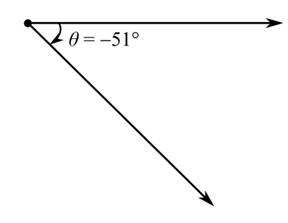Chapter 8.1, Problem 2E### Calculus: An Applied Approach (Min...

10th Edition
Ron Larson
ISBN: 9781305860919

#### Solutions

Chapter
Section### Calculus: An Applied Approach (Min...

10th Edition
Ron Larson
ISBN: 9781305860919
Textbook Problem
9 views

# Finding Coterminal Angles In Exercises 1-6, determine two coterminal angles in degree measure (one positive and one negative) for the angle. See Example 1.To determine

To calculate: The two coterminal angles in degree measure, in which one is positive and another is negative with the help of provided diagram,Explanation

Given Information:

The provided angle is 51°, which is shown below as,

Formula used:

The angle measuring θ has same initial and terminal sides as the angle measuring 360θ, such angles are coterminal angle.

Calculation:

Draw diagram for coterminal angles and provided angle for θ=51°.

From above diagram, let θ+ be the positive coterminal angle and θ be the negative coterminal angle

### Still sussing out bartleby?

Check out a sample textbook solution.

See a sample solution

#### The Solution to Your Study Problems

Bartleby provides explanations to thousands of textbook problems written by our experts, many with advanced degrees!

Get Started

#### Convert the expressions in Exercises 6584 to power form. 23x1.2x2.13

Finite Mathematics and Applied Calculus (MindTap Course List)

#### In Exercises 33-38, rewrite the expression using positive exponents only. 33. (xy)2

Applied Calculus for the Managerial, Life, and Social Sciences: A Brief Approach

#### In problems 23-58, perform the indicated operations and simplify. (x4+3x3x+1)(x2+1)

Mathematical Applications for the Management, Life, and Social Sciences

#### What is the partial fraction form of ?

Study Guide for Stewart's Single Variable Calculus: Early Transcendentals, 8th

#### True or False: is a convergent series.

Study Guide for Stewart's Multivariable Calculus, 8th